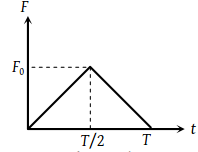A particle of mass m moving with velocity u makes an elastic one dimensional collision with a stationary particle of mass m. They are in contact for a very short time T. Their force of interaction increases from zero to F0 linearly in time T/2, and decreases linearly to zero in further time T/2. The magnitude of F0 is(1) mu/T

(2) 2 mu/T

(3) mu/2T

(4) None of these

Concept Videos :-

#4 | Definition of Momentum
#5 | Conservation of Momentum
#6 | Problems

Concept Questions :-

Linear momentum

(2) In elastic one dimensional collision particle rebounds with same speed in opposite direction

i.e. change in momentum = 2 mu

But Impulse $=F×T=$ Change in momentum

${F}_{0}×T=2mu$${F}_{0}=\frac{2mu}{T}$

Difficulty Level:

• 13%
• 69%
• 16%
• 4%